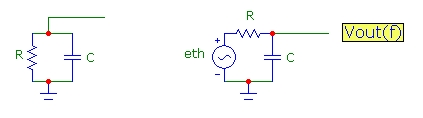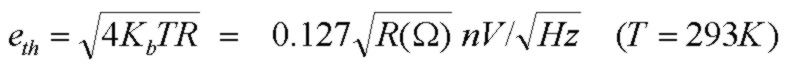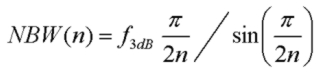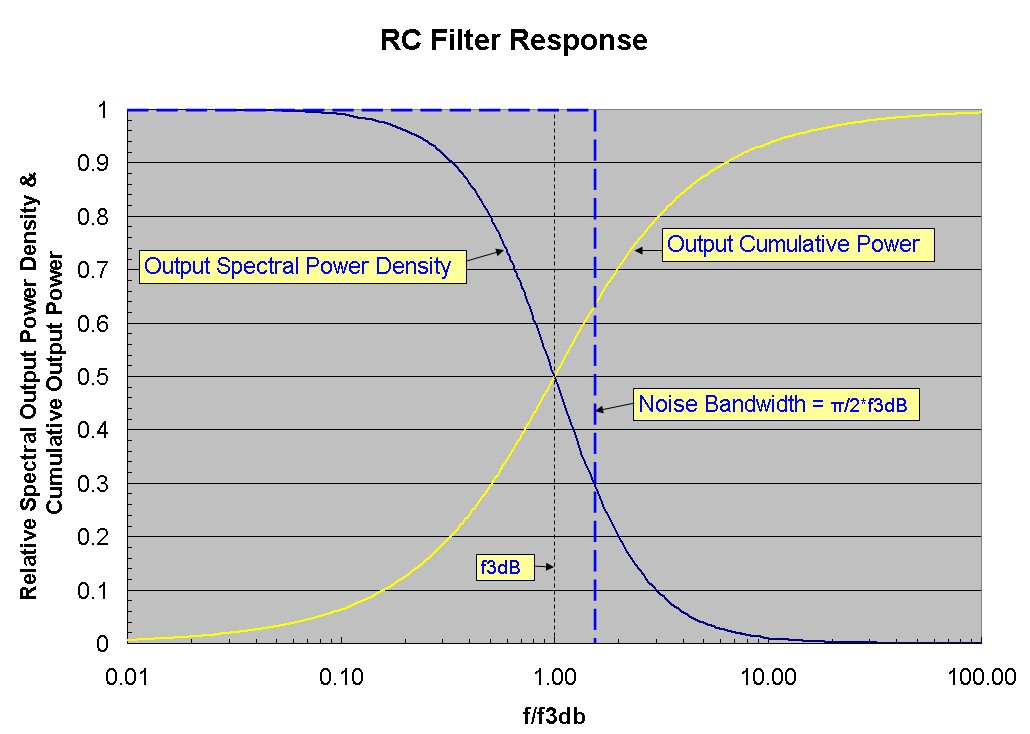# The Concepts of Noise Bandwidth and Cumulative Noise

Jan 17, 2013

This note explains the concept of "noise bandwidth". For simplicity the simple case of the low-pass RC filter is considered with the equivalent circuit for noise shown below:The thermal noise voltage source due to the resistor R driving this simple "single pole" filter has a spectral density in V/√Hz of:eth is independent of frequency to a very good approximation in most cases. The output voltage noise spectral density Vout(f) of this circuit DOES depend on frequency due to the filtering action of the RC combination.

Since this is a low-pass filter, the TOTAL thermal noise voltage, obtained by integrated the square of the output spectral noise voltage density over ALL frequency and taking the square root, will have a finite value because the output voltage spectral density decreases as the frequency is increased. This is the output RMS thermal noise voltage as would be measured with a wide-band AC voltmeter.
The noise bandwidth is the number in Hz which when multiplied by the LOW frequency value of the spectral noise power density at the output (eth^2 in this case) gives the same noise power value as the actual total noise power at the output integrated over the filter transfer function over all frequency. This definition of noise bandwidth also applies to the transfer function of any circuit (not just the simple passive RC filter considered here).

The cumulative output voltage noise at frequency f by comparison is defined as the total output voltage noise INTEGRATED OVER THE ACTUAL FILTER RESPONSE, BUT ONLY TO SOME FINITE FREQUENCY F. Therefore the cumulative output voltage noise tends to the total output voltage noise integrated over all frequency as f -->∞

The chart below for the simple RC filter case will make the definitions clearer. The blue curve is the Output Spectral Power Density Vout(f)^2 in units of V^2/Hz (the curves shown are actually normalized values). The total output RMS voltage noise is obtained by integrating the Spectral Power Density over ALL frequency and taking the square root. The cumulative output power (yellow curve) at frequency f in units of V^2 is obtained by integration of the output spectral power density from zero frequency to finite frequency f. Note that a significant amount of output power occurs at much higher frequency than the f3db point. For example about 90% of the noise power appears within a frequency range up to 7xf3db. The noise bandwidth is indicated by the vertical dashed line. The area under the rectangle defined by the dashed blue lines is the same as the total area under the output spectral power density curve. For this case of the simple RC filter, the noise bandwidth value is exactly π/2*f3db =~ 1.57*f3db where f3db = 1/(2πRC). For flatter and sharper cutoff higher-order filters, the noise bandwidth approaches the f3db value with an infinitely sharp "square" brick-wall filter having identical noise bandwidth and f3db. For example, for maximally-flat Butterworth filters of order n with f3db design values, the noise bandwidth and several useful cases (with n=1 being the simple RC filter case) are:For the RC filter, the total output voltage noise power (integrated over all frequency) is eth^2 * NBW = 4KbTπ/2*1/(2πRC) = KbT/C , a simple result which is independent of the resistance value R. Although the spectral power density of R increases with R, the filter bandwidth decreases as 1/R. The dependences cancel leading to the total noise being independent of R. Note that this TOTAL noise is integrated over all frequency. The cumulative noise up to frequency f will of course depend on the value or R.